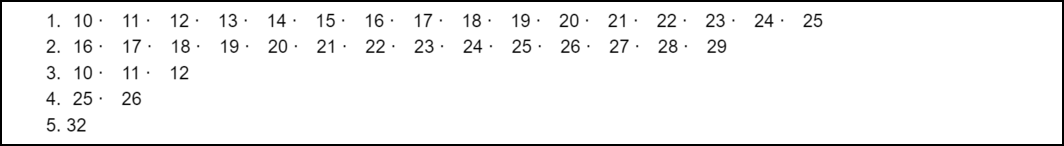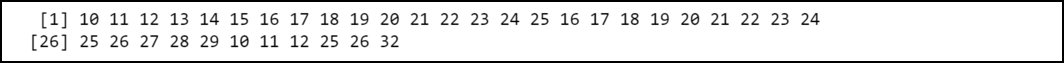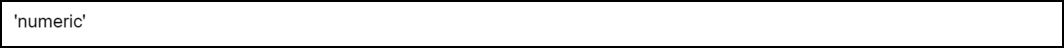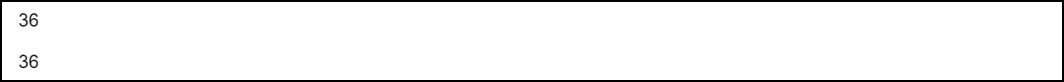GFG App
Open AppBrowser
Continue

# How to Fix in R: (list) object cannot be coerced to type ‘double’

In this article, we are looking towards the way to fix the “(list) object cannot be coerced to type ‘double” error in the R Programming language.

One of the most common errors that a programmer might face in R is:

```Error:
(list) object cannot be coerced to type 'double'```

This error might occur when we try to transform a list of multiple elements into the numeric without using the unlist() function.

## When this error might occur:

Let’s create a list first:

Example:

## R

 `# Make a list` `myList <- ``list``(10:25, 16:29, 10:12, 25:26, 32)`   `# Print the list` `myList`

Output:Output

Now we will convert the list into its numeric equivalent vector using as.numeric() function. The syntax of this function is given below:

Syntax: as.numeric(vect)

Parameter: vect: a vector of characters

## R

 `# Make a list` `myList <- ``list``(10:25, 16:29, 10:12, 25:26, 32)`   `# Convert list to numeric vector` `numeric_vector <- ``as.numeric``(x)`

Output:Output

Since we haven’t used the unlist() function that is why the compiler produced the error: “object that cannot be coerced to type a ‘double’ error message”.

## How to Fix the Error:

This error can be fixed by first bundling the list into a vector using unlist() function and then passing it to the numeric() function. The syntax of the unlist() function is given below:

Syntax: unlist(list)

Parameter: list: It represents a list in R

Return Type: Returns a vector

## R

 `# Make a list` `myList <- ``list``(10:25, 16:29, 10:12, 25:26, 32)`   `# Convert list to numeric` `numeric_vector <- ``as.numeric``(``unlist``(x))`   `# Print the numeric equivalent` `print``(numeric_vector)`

Output:Output

Example:

To verify whether numeric_vector is of type numeric, we can use the class() function. The syntax of the class function is given below:

Syntax: class(x)

Parameter: Here, x represents a R object

Return Type: Returns the type of the passed object i.e, x (vector, list etc)

## R

 `# Make a list` `myList <- ``list``(10:25, 16:29, 10:12, 25:26, 32)`   `# Convert list to numeric` `numeric_vector <- ``as.numeric``(``unlist``(x))`   `# Print the numeric equivalent` `class``(numeric_vector)`

Output:Output

Also, to verify whether myList and numeric_vector contain an equal number of elements we can use a combination of sum() and lengths() function for myList and length() function for numeric_vector.

The syntax of the sum() function is given below:

Syntax: length(x)

Parameter: Here, x represents a R object like a vector or list

Return Type: Returns the number of elements present in x

## R

 `# Make a list` `myList <- ``list``(10:25, 16:29, 10:12, 25:26, 32)`   `# Convert list to numeric` `numeric_vector <- ``as.numeric``(``unlist``(x))`   `# Print the total number of` `# elements in the list` `sum``(``lengths``(myList))`   `# Print the total number of` `# elements in the vector` `length``(numeric_vector)`

Output:Output

My Personal Notes arrow_drop_up